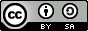## Pages

### Trig 101This semester I ran a partially flipped open source Trigonometry course using a free textbook and youTube videos. To give back to the OER community, I have recreated my blackboard shell here in a single blog post. I am offering all of the worksheets that I have created below in PDF and source DOCX format under a creative commons license (cc-by-sa).  Please feel free to copy anything here, modify, and/or redistribute.

Open Textbook:  Trigonometry by Michael Corral
VideosMathispower4u Trigonometry by James Sousa

End-page Formulas (Trig. cheat sheet): Trigonometry Definitions and Formulas | source ]
Free Graphing Utility: Desmos.com
Free CAS: WolframAlfa

Chapter 1: Right Triangle Trigonometry

1.1 Angles: [ Assignment 1a : source ]

Video notes: Angle Basics
Video notes: Degrees, Minutes, and Seconds
Video notes: Angle Relationships and Types of Triangles
Video notes: The Pythagorean Theorem
Video notes: Converse of the Pythagorean Theorem

1.2 Trigonometric Functions: Assignment 1b : source ]

Video Notes: Introduction to Trigonometric Functions
Video Example: Determine Trigonometric Function Values
Video Example: Trig. Function Values with Hypotenuse Missing

1.3 Applications: Assignment 1c : source ] [ Assignment 1d : source ]

Video Example: Determine the Length of a Side
Video Example: Perimeter of an Equilateral Triangle

1.4 Trigonometric Functions of any Angle: [ Assignment 1e : source ]

Video Examples: Determine the Reference Angle
Video Notes: Introduction to Trig Functions using Angles

1.5 Rotations and Reflections:  [ Assignment 1f source ]

*** *** *** [ Sample Exam #1 | source ] *** *** ***

Chapter 2: General Triangles

2.1 Law of Sines [ Assignment 2a | source ]

Video: The Law of Sines: The Basics
Video: The Law of Sines: The Ambiguous Case
Example: Solve a Triangle Using the Law of Sines

2.2 Law of Cosines Assignment 2b | source ]

Video: The Law of Cosines
Example: Application of the Law of Cosines
Example: Determine an Angle given SSS

2.3 Law of Tangents

2.4 Area of a Triangle Assignment 2c | source ]

Example: Determine the Area of a Triangle Using the Sine Function
Example: Area using Heron's Formula

Chapter 3: Identities

3.1 Basic Identities [ Assignment 3a | source ]

Video: Fundamental Identities
Video: Verifying Identities (good using a slightly different method.)

3.2 Sum and Difference Formulas Assignment 3b | source ]

Video: Sum and Difference Identities for Cosine
Video: Sum and Difference Identities for Sine
Video: Sum and Difference Identities for Tangent

3.3 Double and Half-Angle Formulas Assignment 3c | source ]

Video: Double Angle Identities
Example: Double Angle Example Given Information
Example: Using the Half Angle Formula (use degrees here)
Example: Using Sine of a Half Angle

*** *** *** [ Sample Exam #2 | source ] *** *** ***

4.1 Radian Measure [ Assignment 4a | source ]

4.2 Arc Length Assignment 4b | source ]

Lecture: Arc Length and Area of a Sector

4.3 Area of a Sector Assignment 4c | source ]

Lecture: Arc Length and Area of a Sector

4.4 Circular Motion Assignment 4d | source ]

Lecture: Linear Velocity and Angular Velocity

Chapter 5: Graphing and Inverse Functions

5.1 Graphing Trigonometric Functions [ Assignment 5a | source ]

Video Lecture: Graphing the Sine and Cosine Function
Video Lecture: Graphing the Tangent Function
Video Lecture: Graphing Cosecant and Secant

5.2 Properties of GraphsAssignment 5b | source ]

Video Lecture: Amplitude and period of Sine and Cosine
Video Lecture: Horizontal and Vertical Translations of Sine and Cosine
Video Lecture: Graphing Sine and Cosine with Transformations

5.3 Inverse Trigonometric Functions Assignment 5c | source ]

Video Lecture: Inverse Functions
Video Lecture: Intro. to Inverse Sine, Cosine, and Tangent
Video Examples: Evaluating Expressions involving the Inverse Trig. Functions

*** *** *** [ Sample Exam #3 | source ] *** *** ***

6.1 Solving Trigonometric Equations
Assignment 6a | source ]
Assignment 6b | source ] Assignment 6c | source ]

Video Lecture: Solving Trigonometric Equations I  (Excellent introduction! Linear)
Video Lecture: Solving Trigonometric Equations II (Quadratic examples)
Video Lecture: Solving Trigonometric Equations III (Using Identities first, quadratic formula)
Video Lecture: Solving Trigonometric Equations IV  (Half and multiple angles)

6.2 Numerical Methods in Trigonometry

6.3 Complex Numbers Assignment 6d | source ]

Video Lecture: Introduction to Complex Numbers
Video Lecture: Complex Number Operations
Video Lecture: Trigonometric Form of Complex Numbers
Video Lecture: De Moivre's Theorem
Video Lecture: Nth Roots of a Complex Number

6.4 Polar Coordinates Assignment 6e | source ]

Video Lecture: Introduction to Polar Coordinates
Video Lecture: Converting Polar Equations to Rectangular Equations  (sound is bad)
Video Lecture: Graphing Polar Equations Part I
Video Lecture: Graphing Polar Equations Part II

*** *** *** [ Sample Exam #4 | source ] *** *** ***

*** Sample Final Exam - coming soon ***

Sample Final Exam Questions1.2.